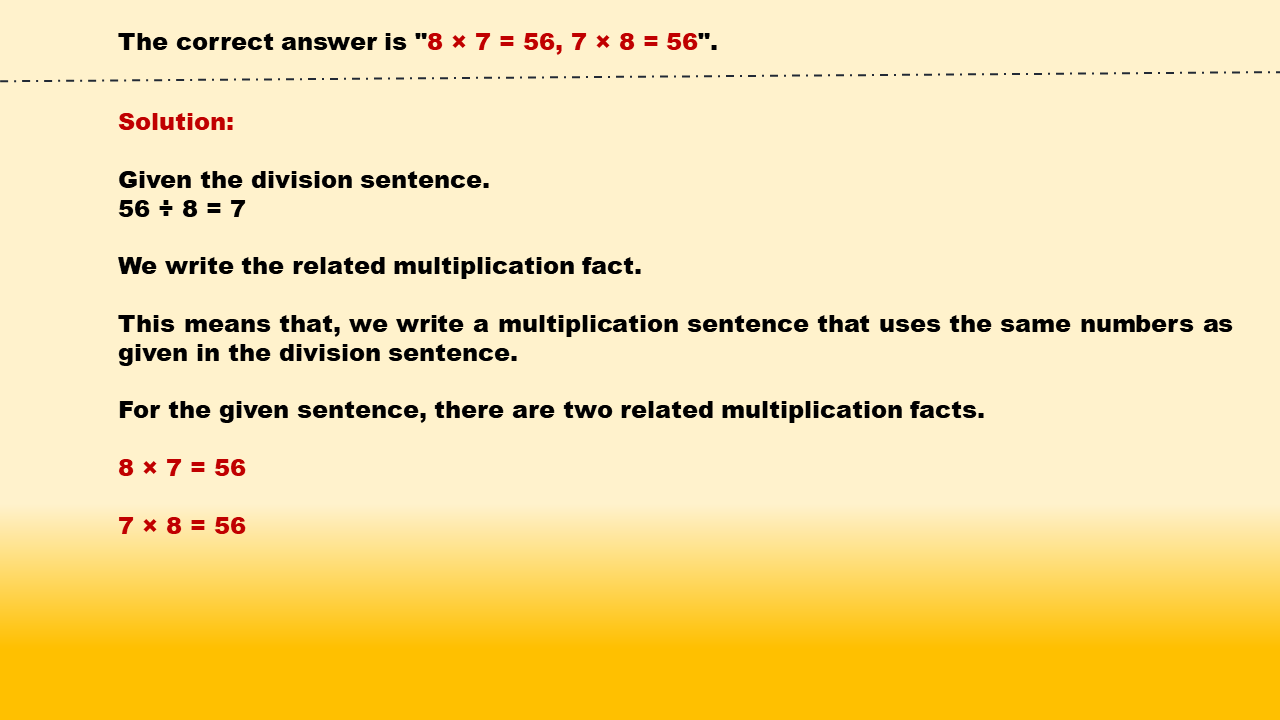1
visibility

Identify related multiplication facts for 56 ÷ 8 = 7.

• 8 × 7 = 56, 7 × 8 = 56

• 8 × 3 = 24, 3 × 8 = 24

• 8 × 6 = 48, 6 × 8 = 48

• 8 × 2 = 16, 2 × 8 = 16